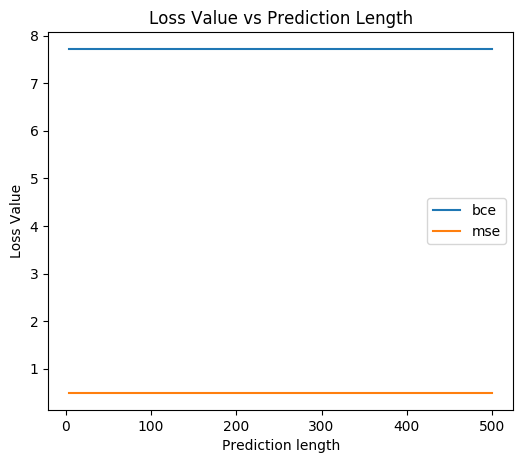# Comparing loss values of different data sizes

While running the hyperparameter optimization of a model, where one of the parameters was the actual data size, I realized that I didn’t know if loss values calculated from different data sizes were comparable. I knew that different loss metrics could not be compared, but I was not sure if different data sizes affected the final value.

Looking at the mean squared error (MSE) and binary cross-entropy (BCE) loss equations:

\begin{align} \text{MSE} &= \frac{1}{N}\sum_{n=1}^{N}\left ( Y_n - \hat{Y}_n \right )^{2} \\ \text{BCE} &= \frac{-1}{N}\sum_{n=1}^{N}\left ( Y_n \log{\hat{Y}_n} + \left ( 1- Y_n \right ) \log\left(1-\hat{Y}_n\right)\right ) \end{align}

Both equations are normalized by the data length $$\textstyle \frac{1}{N}$$, so data of different lengths with the same error proportion should have the same loss value. I tested this by generating prediction $$\textstyle \hat{Y}$$ and actual $$\textstyle Y$$ data of increasing lengths. The predicted data only consisted of zeroes, while the real data was exactly half 0 and half 1.

import tensorflow as tf
tf.enable_eager_execution()
import numpy as np
import matplotlib.pyplot as plt
bce = tf.keras.losses.BinaryCrossentropy()
mse = tf.keras.losses.MeanSquaredError()

# Data Holders
X = np.arange(4,501,2)
Y_bce = np.zeros(len(X))
Y_mse = np.zeros(len(X))

# Generate Loss values
for i,x in enumerate(X):
pred = np.zeros(x)
real = np.zeros(x)
real[::2] = 1 # Every other value == 1
Y_bce[i] = bce(real, pred).numpy()
Y_mse[i] = mse(real, pred).numpy()
plt.figure(figsize=(6,5), dpi=100)
plt.plot(X,Y_bce, label="bce")
plt.plot(X,Y_mse, label="mse")
plt.xlabel("Prediction length")
plt.ylabel("Loss Value")
plt.title("Loss Value vs Prediction Length")
plt.legend()
plt.show()


The resulting figure shows that the loss value for both functions is stable for different lengths when the error proportion is the same.This confirms that loss values calculated from data of different sizes can be compared in a hyperparameter optimization, and I can continue with my work again.

22 Jan 2020